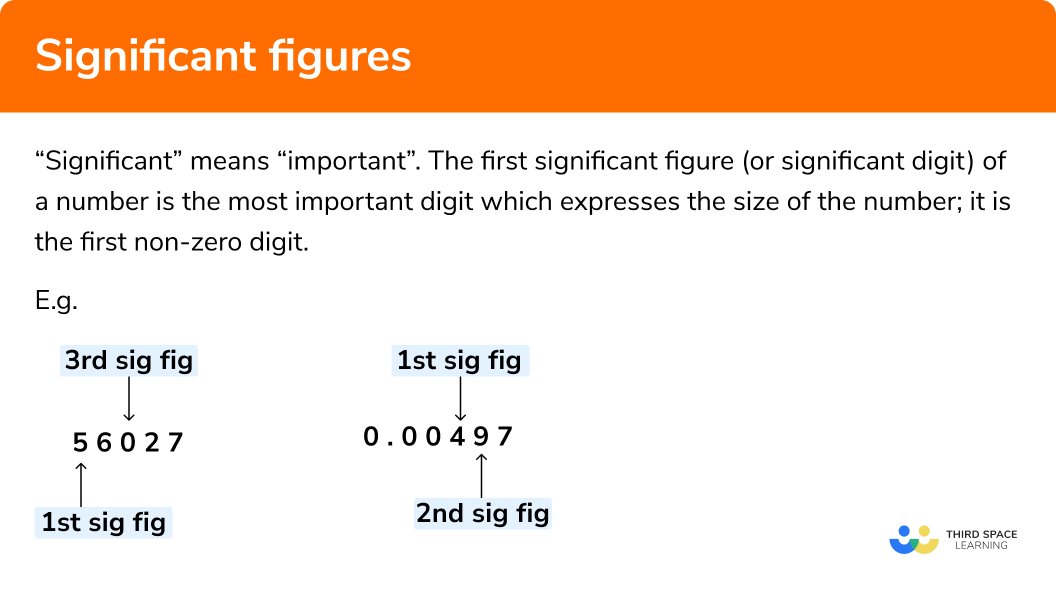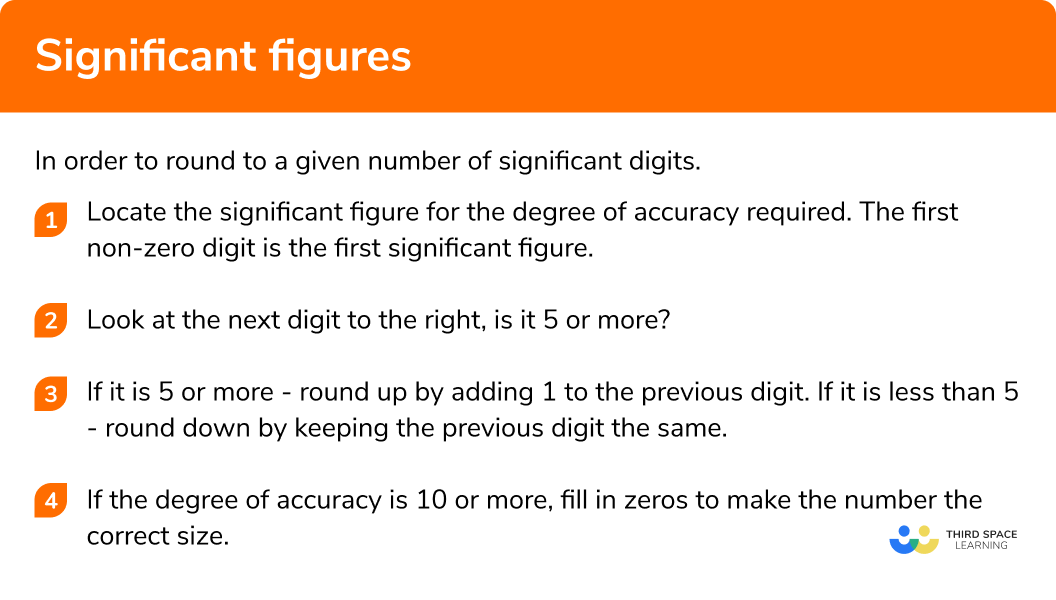GCSE Maths Number Rounding Numbers

Significant Figures

# Significant Figures

Here we will learn about significant figures including how to round numbers to one significant figure, two significant figures and three significant figures.

There are also rounding to significant figures worksheets based on Edexcel, AQA and OCR exam questions, along with further guidance on where to go next if you’re still stuck.

## What are significant figures?

Significant figures are the digits in a number that contribute to the accuracy of it.
We start counting significant figures at the first non-zero digit of a number which is called the first significant figure, the next digit is then called the second significant figure and so on.

A significant figure could be to the left of the decimal point or the right of the decimal point.

E.g.

Rounding numbers to significant figures (often abbreviated to sig figs or s.f.) is similar to rounding to a number of decimal places, units, tens, hundreds and thousands but in this case we need to find the appropriate significant figure to give the correct degree of accuracy.

### What are significant figures?## How to round to significant figures

In order to round to a given number of significant digits:

1. Locate the significant figure for the degree of accuracy required. The first non-zero digit is the first significant figure.
2. Look at the next digit to the right. Is it 5 or more?
3. If it is 5 or more – round up by adding 1 to the previous digit.
If it is less than 5 – round down by keeping the previous digit the same.
If the degree of accuracy is 10 or more, fill in zeros to make the number the correct size.

### Explain how to round to a given number of significant digits in 3 steps## Rounding to significant figure examples

### Example 1: rounding to one significant figure

Round 3692 to one significant figure 1 s.f.

1. Locate the significant figure for the degree of accuracy required. The first non-zero digit is the first significant figure.

3692

The first non-zero digit is the 3, therefore 3 is the first significant figure.

2Look at the next digit to the right. Is it 5 or more?

3¦692

6 is more than 5.

3If it is 5 or more – round up by adding 1 to the previous digit. If it is less than 5 – round down by keeping the previous digit the same. If the degree of accuracy is 10 or more, fill in zeros to make the number the correct size.

As 6 is more than 5, we round up. Adding 1 to the 3, gives us 4. The first significant figure represents thousands, so we must add three zeros to make it the correct size.

3692 is 4000 to 1 s.f.

### Example 2: rounding to two significant figures

Round 0.07039 to two significant figures.

0.07039

The first non-zero digit is the 7 which is the first significant figure, therefore the 0, at the thousandths place, is the second significant figure.

0.070¦39

3 is less than 5.

As 3 is less than 5, we round down.

0.07039 is 0.070 to 2 s.f.

It is important to keep the zero after the seven as it must be given to two significant figures.

### Example 3: rounding to three significant figures

Round 24.753 to three significant figures 3 s.f.

24.753

The first non-zero digit is the 2 which is the first significant figure, therefore the 7, at the tenths place, is the third significant figure.

24.7¦53

The digit to the right is 5.

As it is a 5, we round up.

24.7 is 24.8 to 3 s.f.

### Common misconceptions

• Assuming the first zeros are significant

A common error is to think the first zeros in a number like 0.00467 are significant. These are called leading zeros and do not tell us the size of the number. The first non-zero digit is 4, this tells us that the number is roughly 4 thousandths.

• Assuming all zeros are not significant

A common error is to think that all zeros in a number like 9006 are not significant. Once the first significant figure has been located, all digits after are significant. Otherwise we might round to the incorrect number of significant figures.

• Leaving out zeros to make the number the correct size

It is important to remember to fill in the zeros if the degree of accuracy is more than 10. A common mistake when rounding a number like 378 to two significant figures is to say it is 38 and not 380.

Significant figures are part of our series of lessons to support revision on rounding numbers. You may find it helpful to start with the main rounding numbers lesson for a summary of what to expect, or use the step by step guides below for further detail on individual topics. Other lessons in this series include:

### Practice significant figures questions

Round 76340 to one significant figure.

870000800008000The first significant figure is the 7 . The next digit to the right is 6 , which is bigger than 5 , so we round up. Adding 1 to 7 gives us 8 and filling in the zeros gives us 80000 .

2. Round 0.3897 to one significant figure.

0.30.401The first significant figure is the 3 . The next digit to the right is 8 , which is bigger than 5 , so we round up. Adding 1 to 3 gives us 4 therefore the answer is 0.4 .

3. Round 26460 to two significant figures.

25000270002626000The first significant figure is 2 and the second is 6 . The next number to the right is 4 , which is less than 5 , so we round down. The 6 remains the same and filling in the zeros gives us 26000 .

4. Round 78.56 to two significant figures.

78.6787980The first significant figure is 7 and the second is 8 . The next number to the right is 5 , so we round up. Adding one to the 8 gives us 9 and therefore we have 79 .

5. Round 4.7041294 to three significant figures.

4.704.7044.715.70The third significant figure is 0 . The next figure to the right is 4 , which is less than 5 . Therefore we round down and the 0 remains the same. The answer is 4.70 to 3 s.f.

6. Round 0.0051489 to three significant figures.

0.0050.005150.005140.00525The first significant figure is 5 and the third significant figure is 4 . The next number to the right is 8 , which is bigger than 5 , so we round up. Adding 1 to the 4 gives us 5 and therefore we have 0.00515 .

### Significant figures GCSE questions

1. (a) Write 467983 correct to two significant figures.

(b) Write 0.03887 correct to one significant figure.

(c) Write 60.7328 correct to three significant figures.

(3 marks)

470000

(1)

0.04

(1)

60.7

(1)

2. Sam was finding the area of a compound shape. His calculated result was 60942.937 cm2. He needs to round this area to two significant figures, choose the correct answer.

60900 cm2    61000 cm2    60942.94 cm2    60000 cm2

(1 mark)

61000cm2

3. (a) Use a calculator to work out \frac{\sqrt{6.79}}{3.72-2.81} . Write down all of the digits on your calculator screen.

(3 marks)

(a) Finding intermediate results of 2.605 … or 0.91 from the subtraction seen.

(1)

Final result of 2.863475653

(1)

(b) Rounded correctly to 2.86

(1)

## Learning checklist

You have now learned how to:

• Apply and interpret limits of accuracy when rounding

## Still stuck?

Prepare your KS4 students for maths GCSEs success with Third Space Learning. Weekly online one to one GCSE maths revision lessons delivered by expert maths tutors.

Find out more about our GCSE maths tuition programme.